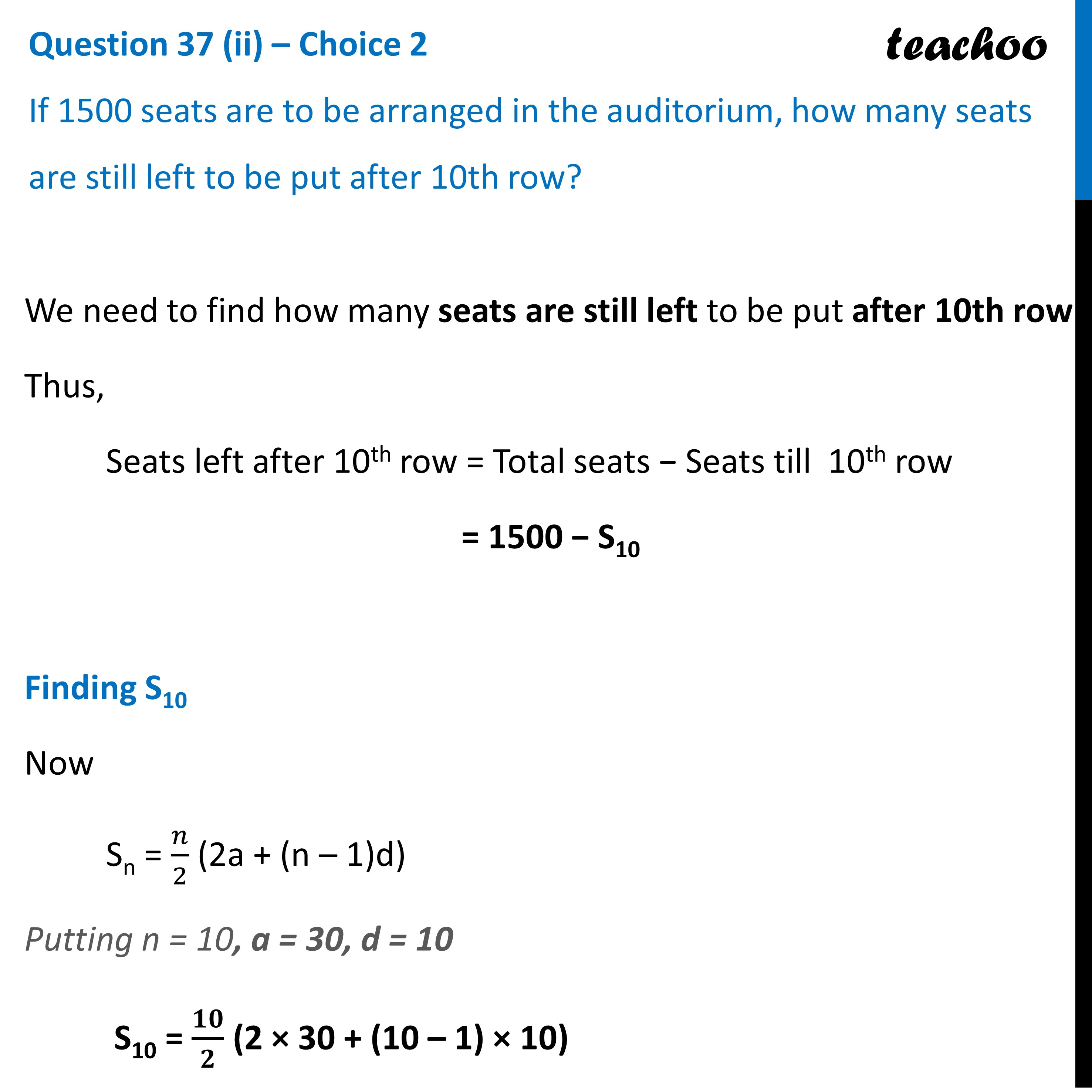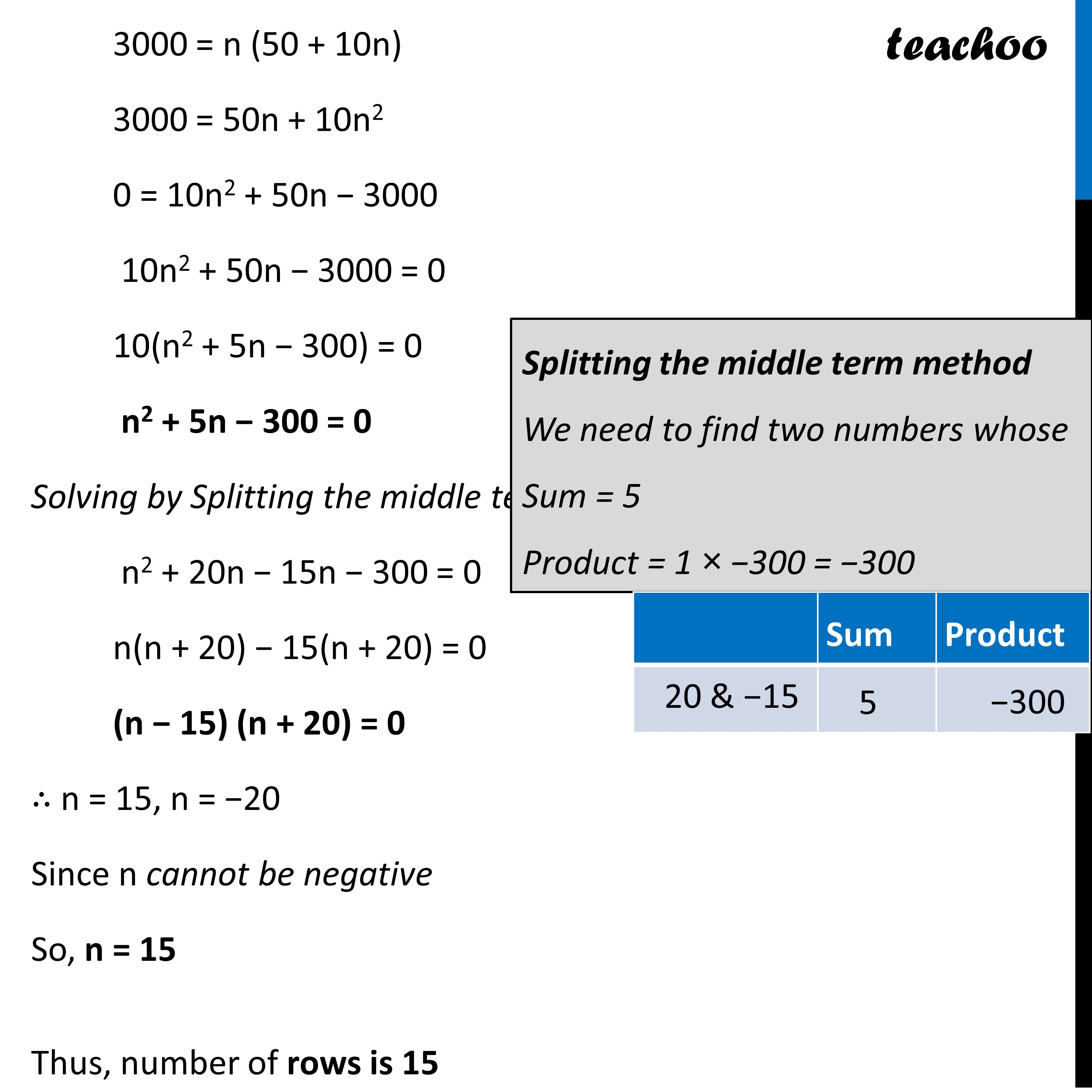CBSE Class 10 Sample Paper for 2023 Boards - Maths Standard

Class 10
Solutions of Sample Papers for Class 10 Boards

## For 1500 seats in the auditorium, how many rows need to be there?Learn in your speed, with individual attention - Teachoo Maths 1-on-1 Class

### Transcript

Question 37 (ii) – Choice 1 For 1500 seats in the auditorium, how many rows need to be there? Since Total seats = 1500 Given Sn = 1500, We need to find number of rows, i.e., we need to find n Now Sn = 𝑛/2 (2a + (n – 1)d) Putting Sn = 1500, a = 30, d = 10 1500 = 𝒏/𝟐 (2 × 30 + (n – 1) × 10) 1500 × 2 = n (60 + 10(n − 1)) 3000 = n (60 + 10n − 10) 3000 = n (50 + 10n) 3000 = 50n + 10n2 0 = 10n2 + 50n − 3000 10n2 + 50n − 3000 = 0 10(n2 + 5n − 300) = 0 n2 + 5n − 300 = 0 Solving by Splitting the middle term n2 + 20n − 15n − 300 = 0 n(n + 20) − 15(n + 20) = 0 (n − 15) (n + 20) = 0 ∴ n = 15, n = −20 Since n cannot be negative So, n = 15 Thus, number of rows is 15